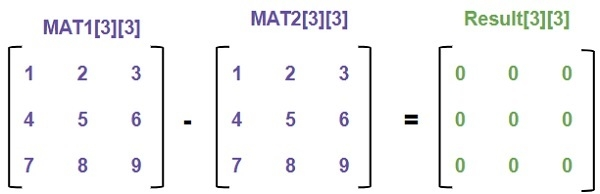# C Program for subtraction of matrices

Given two matrices MAT1[row][column] and MAT2[row][column] we have to find the difference between two matrices and print the result obtained after subtraction of two matrices. Subtraction of two matrices are MAT1[n][m] – MAT2[n][m].For subtraction the number of rows and columns of both matrices should be same.

## Example

Input:
MAT1[N][N] = { {1, 2, 3},
{4, 5, 6},
{7, 8, 9}}
MAT2[N][N] = { {9, 8, 7},
{6, 5, 4},
{3, 2, 1}}
Output:
-8 -6 -4
-2 0 2
4 6 8

Approach used below is as follows

We will iterate both matrix for every row and column and subtract the values of mat2[][] from mat1[][] and store the result in a result[][] where row and column remain same for all the matrices.

## Algorithm

In fucntion void subtract(int MAT1[][N], int MAT2[][N], int RESULT[][N])
Step 1-> Declare 2 integers i, j
Step 2-> Loop For i = 0 and i < N and i++
Loop For j = 0 and j < N and j++
Set RESULT[i][j] as MAT1[i][j] - MAT2[i][j]
In function int main()
Step 1-> Declare a matrix MAT1[N][N] and MAT2[N][N]
Step 2-> Call function subtract(MAT1, MAT2, RESULT);
Step 3-> Print the result

## Example

Live Demo

#include <stdio.h>
#define N 3
// This function subtracts MAT2[][] from MAT1[][], and stores
// the result in RESULT[][]
void subtract(int MAT1[][N], int MAT2[][N], int RESULT[][N]) {
int i, j;
for (i = 0; i < N; i++)
for (j = 0; j < N; j++)
RESULT[i][j] = MAT1[i][j] - MAT2[i][j];
}
int main() {
int MAT1[N][N] = { {1, 2, 3},
{4, 5, 6},
{7, 8, 9}
};
int MAT2[N][N] = { {9, 8, 7},
{6, 5, 4},
{3, 2, 1}
};
int RESULT[N][N]; // To store result
int i, j;
subtract(MAT1, MAT2, RESULT);
printf("Resultant matrix is ");
for (i = 0; i < N; i++) {
for (j = 0; j < N; j++)
printf("%d ", RESULT[i][j]);
printf("");
}
return 0;
}

## Output

If run the above code it will generate the following output −

Resultant matrix is
-8 -6 -4
-2  0  2
4  6  8